Home > CC4 > Chapter 11 > Lesson 11.2.5 > Problem11-109

11-109.
1.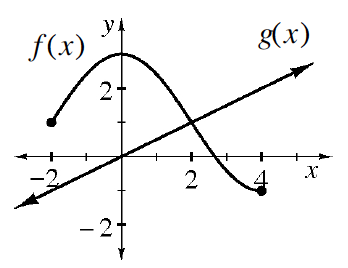Examine the graph of functions f(x) and g(x) at right. Use the graph to approximate the values below (if possible). Homework Help ✎

2.  a. f(0) b. g(4) c. f(2) d. g(0) e. g(2) f. f(5) g. x such that f(x) = g(x)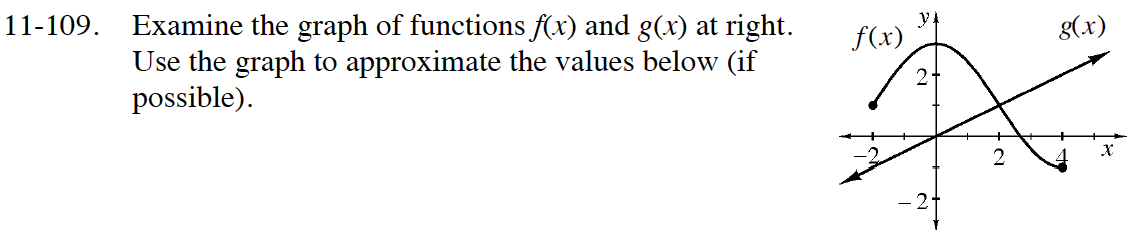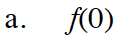When x is 0 in the relation f(x), what is y?

f(0) = 3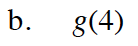When x is 4 in the relation g(x), what is y?

g(4) = 2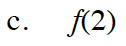See the hint for part (a).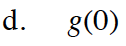See the hint for part (b).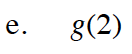See the hint for part (b).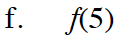Is there a y-value for f(x) when x is 5?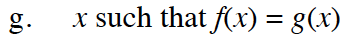For what x value are the two functions equal?